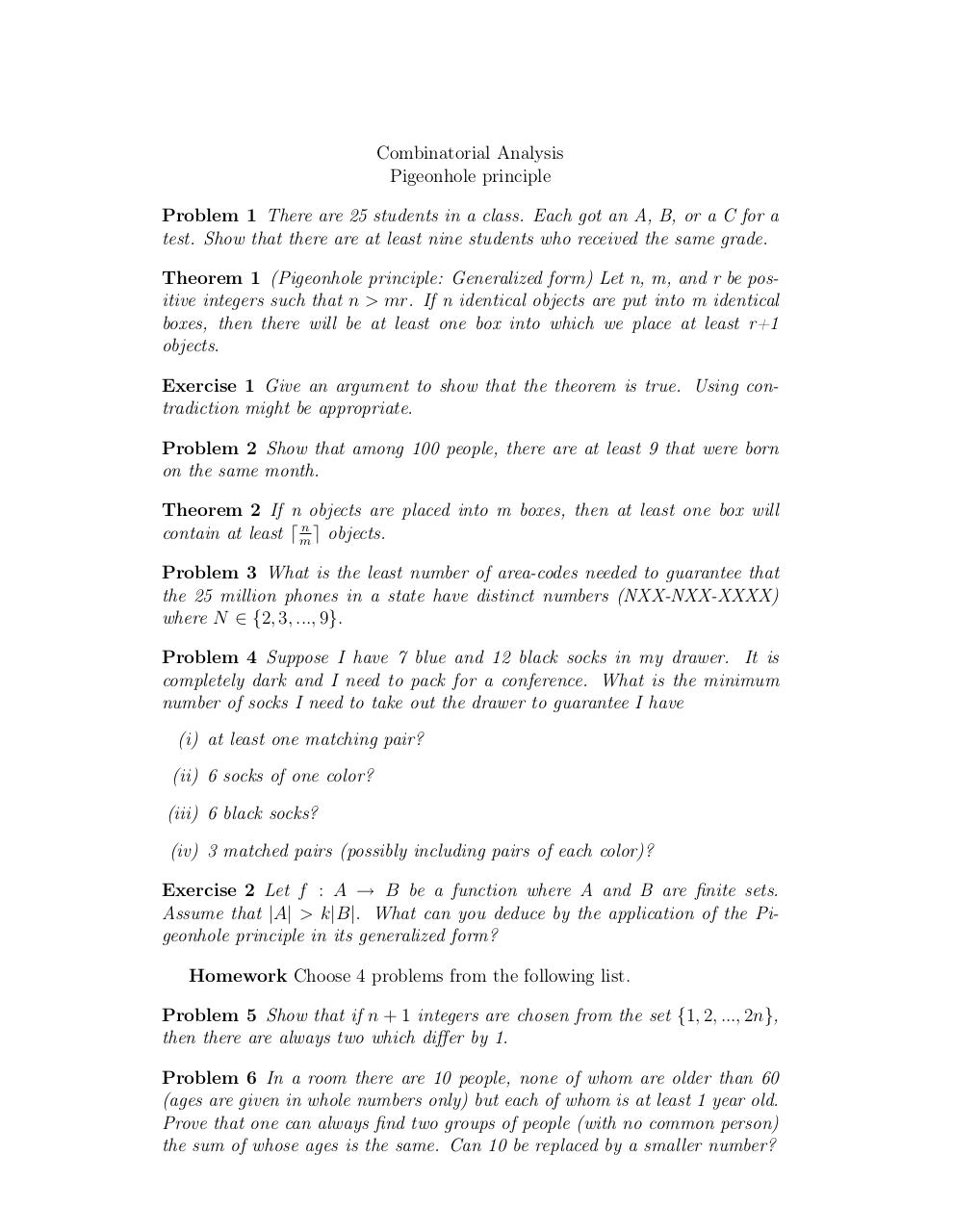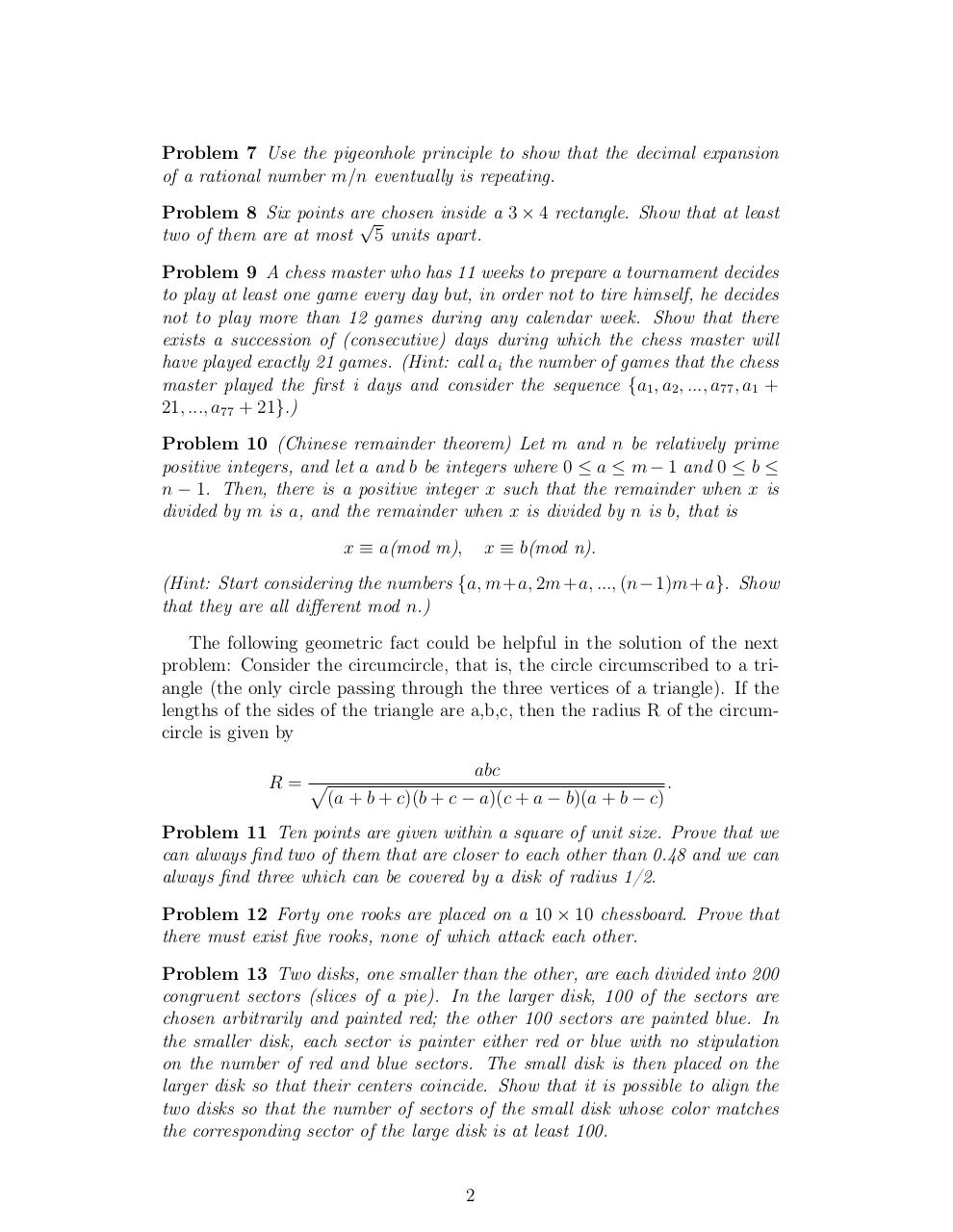# Day 3 Pigeonhole .pdf

### File information

Original filename: Day 3-Pigeonhole.pdf

This PDF 1.4 document has been generated by TeX / pdfTeX-1.40.3, and has been sent on pdf-archive.com on 24/01/2014 at 06:06, from IP address 169.231.x.x. The current document download page has been viewed 1115 times.
File size: 101 KB (2 pages).
Privacy: public file

Day 3-Pigeonhole.pdf (PDF, 101 KB)

### Document preview

Combinatorial Analysis
Pigeonhole principle
Problem 1 There are 25 students in a class. Each got an A, B, or a C for a
test. Show that there are at least nine students who received the same grade.
Theorem 1 (Pigeonhole principle: Generalized form) Let n, m, and r be positive integers such that n &gt; mr. If n identical objects are put into m identical
boxes, then there will be at least one box into which we place at least r+1
objects.
Exercise 1 Give an argument to show that the theorem is true. Using contradiction might be appropriate.
Problem 2 Show that among 100 people, there are at least 9 that were born
on the same month.
Theorem 2 If n objects are placed into m boxes, then at least one box will
n
e objects.
contain at least d m
Problem 3 What is the least number of area-codes needed to guarantee that
the 25 million phones in a state have distinct numbers (NXX-NXX-XXXX)
where N ∈ {2, 3, ..., 9}.
Problem 4 Suppose I have 7 blue and 12 black socks in my drawer. It is
completely dark and I need to pack for a conference. What is the minimum
number of socks I need to take out the drawer to guarantee I have
(i) at least one matching pair?
(ii) 6 socks of one color?
(iii) 6 black socks?
(iv) 3 matched pairs (possibly including pairs of each color)?
Exercise 2 Let f : A → B be a function where A and B are finite sets.
Assume that |A| &gt; k|B|. What can you deduce by the application of the Pigeonhole principle in its generalized form?
Homework Choose 4 problems from the following list.
Problem 5 Show that if n + 1 integers are chosen from the set {1, 2, ..., 2n},
then there are always two which differ by 1.
Problem 6 In a room there are 10 people, none of whom are older than 60
(ages are given in whole numbers only) but each of whom is at least 1 year old.
Prove that one can always find two groups of people (with no common person)
the sum of whose ages is the same. Can 10 be replaced by a smaller number?

Problem 7 Use the pigeonhole principle to show that the decimal expansion
of a rational number m/n eventually is repeating.
Problem 8 Six points are
√ chosen inside a 3 × 4 rectangle. Show that at least
two of them are at most 5 units apart.
Problem 9 A chess master who has 11 weeks to prepare a tournament decides
to play at least one game every day but, in order not to tire himself, he decides
not to play more than 12 games during any calendar week. Show that there
exists a succession of (consecutive) days during which the chess master will
have played exactly 21 games. (Hint: call ai the number of games that the chess
master played the first i days and consider the sequence {a1 , a2 , ..., a77 , a1 +
21, ..., a77 + 21}.)
Problem 10 (Chinese remainder theorem) Let m and n be relatively prime
positive integers, and let a and b be integers where 0 ≤ a ≤ m − 1 and 0 ≤ b ≤
n − 1. Then, there is a positive integer x such that the remainder when x is
divided by m is a, and the remainder when x is divided by n is b, that is
x ≡ a(mod m),

x ≡ b(mod n).

(Hint: Start considering the numbers {a, m+a, 2m+a, ..., (n−1)m+a}. Show
that they are all different mod n.)
The following geometric fact could be helpful in the solution of the next
problem: Consider the circumcircle, that is, the circle circumscribed to a triangle (the only circle passing through the three vertices of a triangle). If the
lengths of the sides of the triangle are a,b,c, then the radius R of the circumcircle is given by
abc
.
R= p
(a + b + c)(b + c − a)(c + a − b)(a + b − c)
Problem 11 Ten points are given within a square of unit size. Prove that we
can always find two of them that are closer to each other than 0.48 and we can
always find three which can be covered by a disk of radius 1/2.
Problem 12 Forty one rooks are placed on a 10 × 10 chessboard. Prove that
there must exist five rooks, none of which attack each other.
Problem 13 Two disks, one smaller than the other, are each divided into 200
congruent sectors (slices of a pie). In the larger disk, 100 of the sectors are
chosen arbitrarily and painted red; the other 100 sectors are painted blue. In
the smaller disk, each sector is painter either red or blue with no stipulation
on the number of red and blue sectors. The small disk is then placed on the
larger disk so that their centers coincide. Show that it is possible to align the
two disks so that the number of sectors of the small disk whose color matches
the corresponding sector of the large disk is at least 100.

2#### HTML Code

Copy the following HTML code to share your document on a Website or Blog

#### QR Code### Related keywords